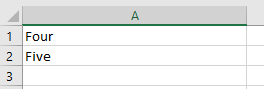# VBA Split Function – Split String of Text into Array

## VBA Split Function

The VBA Split function splits a string of text into substrings based on a specific delimiter character (e.g. a comma, space, or a colon). It is easier to use than writing code to search for the delimiters in the string and then extracting the values.

It could be used if you are reading in a line from a Comma-Separated Value (CSV file) or you have a mailing address that is all on one line, but you want to see it as multiple lines.

The syntax is:

``Split expression, delimiter[optional], limit[optional], compare[optional]``

The VBA Split function has four parameters:

• Expression – The string of text that you wish to split into different parts.
• Delimiter (optional)– string or non-printable character – Defines the delimiter character that is going to be used for the split. If no delimiter character is provided then the default of a space is used.
• Limit (optional) – number – Defines how many splits will be made. If blank, all available splits will be made within the string. If it is set to 1, then no splits will be made.  Basically, it enables you to separate out a specific number of values starting at the beginning of the string e.g. where the string is very long and you only need the first three splits.
• Compare (optional) – If your delimiter is a text character then this is used to toggle whether the delimiter is case-sensitive or not. Values are vbBinaryCompare ( case-senstiive) and vbTextCompare (not-case sensitive).

The split function always returns an array.

Simple Example of the Split Function

``````Sub SplitExample()
'Define variables
Dim MyArray() As String, MyString As String, I As Variant
'Sample string with space delimiters
MyString = "One Two Three Four"
'Use Split function to divide up the component parts of the string
MyArray = Split(MyString)
'iterate through the array created to show each value
For Each I In MyArray
MsgBox I
Next I
End Sub
``````

In this example, no delimiter is specified because all the words have a space in between them, so the default delimiter (space) can be used.

The array has no dimensions, and is set as a string. The variable I, which is used in the For…Next loop must be dimensioned as a variant.

When this code is run, it will show four message boxes, one for each of the splits e.g. One, Two, Three. Four.

Note that if there is a double space between the words in the string, this will be evaluated as a split, although with nothing in it. This may not be the result that you want to see.

You can fix this problem by using the Replace function to replace any double spaces with a single space:

``MyString = Replace(MyString, "  ", " ")``

A trailing or leading space can also cause problems by producing an empty split.  These are often very difficult to see. You can remove these extraneous spaces by using the Trim Function:

``MyString = Trim(MyString)``

### Using the Split Function with a Delimiter Character

We can use a delimiter of a semi-colon (;). This is frequently found in email address strings to separate the addresses. You may have an email sent to you which is shared with a number of colleagues and you want to see a list in your worksheet of who it has gone to. You can easily copy the email addresses from the email ‘To’ or ‘Copy’ boxes and into your code.

``````Sub SplitBySemicolonExample()
'Define variables
Dim MyArray() As String, MyString As String, I As Variant, N As Integer
'Sample string with semi colon delimiters
MyString = "john@myco.com;jane@myco.com;bill@myco.com;james@myco.com"
'Use Split function to divide up the component parts of the string
MyArray = Split(MyString, ";")
'Clear the worksheet
ActiveSheet.UsedRange.Clear
'iterate through the array
For N = 0 To UBound(MyArray)
'Place each email address into the first column of the worksheet
Range("A" & N + 1).Value = MyArray(N)
Next N
End Sub
``````

Note that a For…Next loop is used to iterate through the array. The first element in the array always starts at zero, and the Upper Bound Function is used to get the maximum number of elements.

After running this code, your worksheet will look like this: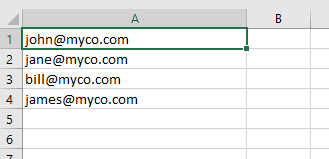### Using a Limit Parameter in a Split Function

The limit parameter allows a specific number of splits to be done from the start of the string.  Unfortunately, you cannot provide a start position or a range of splits to be done, so it is fairly basic. You can build your own VBA code to create a function to do this, and this will be explained later in this article.

``````Sub SplitWithLimitExample()
'Create variables
Dim MyArray() As String, MyString As String, I As Variant, N As Integer
'Sample string with comma delimiters
MyString = "One,Two,Three,Four,Five,Six"
'Use Split function to divide up the component parts of the string
MyArray = Split(MyString, ",", 4)
'Clear the worksheet
ActiveSheet.UsedRange.Clear
'Iterate through the array
For N = 0 To UBound(MyArray)
'Place each split into the first column of the worksheet
Range("A" & N + 1).Value = MyArray(N)
Next N
End Sub
``````

After you run this code, your worksheet will look like this: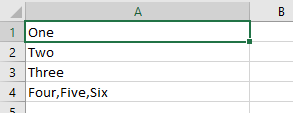Only the first three split values are shown separately. The later three values are shown as one long string and do not get split.

If you choose a limit value that is greater than the number of delimiters within a string, this will not produce an error.  The string will be split into all its component parts as if the limit value had not been provided.

### Using the Compare Parameter in a Split Function

The Compare parameter determines if the delimiter is case-sensitive or not. This is not applicable if the delimiters are commas, semi-colons, or colons.

Note: Instead, you can always place Option Compare Text at the top of your module to eliminate case-sensitivity for the entire module.

``````Sub SplitByCompareExample()
'Create variables
Dim MyArray() As String, MyString As String, I As Variant, N As Integer
'Sample string with X delimiters
MyString = "OneXTwoXThreexFourXFivexSix"
'Use Split function to divide up the component parts of the string
MyArray = Split(MyString, "X", , vbBinaryCompare)
'Clear the worksheet
ActiveSheet.UsedRange.Clear
'iterate through the array
For N = 0 To UBound(MyArray)
'Place each split into the first column of the worksheet
Range("A" & N + 1).Value = MyArray(N)
Next N
End Sub
``````

In this example, the string to be split uses the ‘X’ character as a delimiter. However, in this string, there is a mixture of upper and lower case ‘X’ characters. The Compare parameter in the Split function uses an upper case ‘X’ character.

If the Compare parameter is set to vbBinaryCompare, then the lower case ‘x’ characters will be ignored and your worksheet will look like this: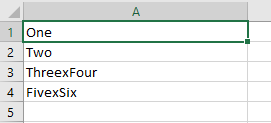If the Compare parameter is set to vbTextCompare, then the lower case ‘x’ characters will be used in the split and your worksheet will look like this: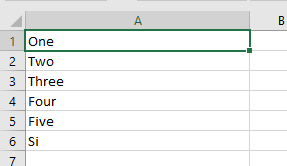Note that the value at cell A6 is truncated because it contains a lower case ‘x’ character. Because the split is not case sensitive, any delimiter which forms part of a sub string will cause a split to happen.

This is an important point to bear in mind when using a text delimiter and vbTextCompare. You can easily end up with the wrong result.

### Using Non-Printable Characters as the Delimiter Character

You can use non printable characters as the delimiter, such as a carriage return (a line break).

Here we use the vbCr to specify a carriage return.

``````Sub SplitByNonPrintableExample()
'Create variables
Dim MyArray() As String, MyString As String, I As Variant, N As Integer
'Sample string with carriage return delimiters
MyString = "One" & vbCr & "Two" & vbCr & "Three" & vbCr & "Four" & vbCr & "Five" & vbCr & "Six"
'Use Split function to divide up the component parts of the string
MyArray = Split(MyString, vbCr, , vbTextCompare)
'Clear the worksheet
ActiveSheet.UsedRange.Clear
'Iterate through the array
For N = 0 To UBound(MyArray)
'Place each split into the first column of the worksheet
Range("A" & N + 1).Value = MyArray(N)
Next N
End Sub
``````

In this example, a string is built up using vbCr (carriage return character) as the delimiter.

When this code is run, your worksheet will look like this: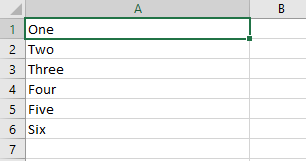Stop searching for VBA code online. Learn more about AutoMacro - A VBA Code Builder that allows beginners to code procedures from scratch with minimal coding knowledge and with many time-saving features for all users!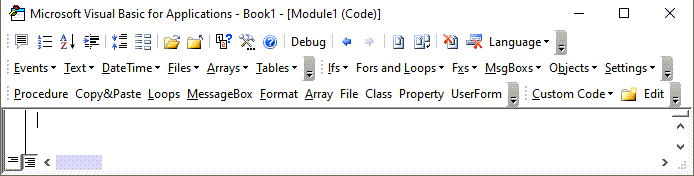### Using the Join Function to Reverse a Split

The Join function will re-join all the elements of an array, but using a specified delimiter. If no delimiter character is specified then a space will be used.

``````Sub JoinExample()
'Create variables
Dim MyArray() As String, MyString As String, I As Variant, N As Integer
Dim Target As String
'Sample string with comma delimiters
MyString = "One,Two,Three,Four,Five,Six"
'Place MyString at cell A1
Range("A1").Value = MyString
'Use Split function to divide up the component parts of the string
MyArray = Split(MyString, ",")
'Use Join function to re-create the original string using a semi colon delimiter
Target = Join(MyArray,”;”)
'Place the result string at cell A2
Range("A2").Value = Target
End Sub
``````

This code splits a string with comma delimiters into an array, and joins it back together using semi-colon delimiters.

After running this code your worksheet will look like this: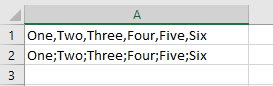Cell A1 has the original string with comma delimiters, and cell A2 has the new joined string with semi-colon delimiters.

### Using the Split Function to do a Word Count

Bearing in mind that a string variable in Excel VBA can be up to 2Gb long, you can use the split function to do word count in a piece of text.  Obviously, Microsoft Word does it automatically, but this could be useful for a simple text file or text copied from another application.

``````Sub NumberOfWordsExample()
'Create variables
Dim MyArray() As String, MyString As String
'Sample string with space delimiters
MyString = "One Two Three Four Five Six"
'Remove any double spaces
MyString = Replace(MyString, "  ", " ")
'Remove any leading or trailing spaces
MyString = Trim(MyString)
'Use Split function to divide up the component parts of the string
MyArray = Split(MyString)
'Show number of words using the UBound function
MsgBox "Number of Words " & UBound(MyArray) + 1
End Sub
``````

One of the dangers of this word count code is that it will be thrown by double spaces and leading and trailing spaces.  If these are present, they will be counted as extra words, and the word count will end up as inaccurate.

The code uses the Replace and Trim functions to remove these extra spaces.

The final code line displays the number of words found by using the UBound function to get the maximum element number of the array and then incrementing it by 1. This is because the first array element begins at zero.

### Splitting an Address into Worksheet Cells

Mail addresses are often long strings of text with comma delimiters. You may well want to split each part of the address into a separate cell.

``````Sub AddressExample()
'Create variables
Dim MyArray() As String, MyString As String, N As Integer
'Set up string with Microsoft Corporation Address
MyString = "Microsoft Corporation, One Microsoft Way, Redmond, WA 98052-6399 USA"
'Use the split function to divide the string using a comma delimiter
MyArray = Split(MyString, ",")
'Clear the worksheet
ActiveSheet.UsedRange.Clear
'iterate through the array
For N = 0 To UBound(MyArray)
'Place each split into the first column of the worksheet
Range("A" & N + 1).Value = MyArray(N)
Next N
End Sub
``````

Running this code will use the comma delimiter to put each line of the address into a separate cell: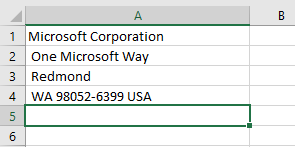If you only wanted to return the zip code (last array element), then you could use the code:

``````Sub AddressZipCodeExample()
'Create variables
Dim MyArray() As String, MyString As String, N As Integer, Temp As String
'Set up string with Microsoft Corporation Address
MyString = "Microsoft Corporation, One Microsoft Way, Redmond, WA 98052-6399 USA"
'Use the split function to divide the string using a comma delimiter
MyArray = Split(MyString, ",")
'Clear the worksheet
ActiveSheet.UsedRange.Clear
'Put the Zip Code at cell A1
Range("A1").Value = MyArray(UBound(MyArray))
End Sub
``````

This will only use the last element in the array, which is found by using the UBound function.

On the other hand, you may wish to see all the lines in one cell so that they can be printed onto an address label:

``````Sub AddressExample()
'Create variables
Dim MyArray() As String, MyString As String, N As Integer, Temp As String
'Set up string with Microsoft Corporation Address
MyString = "Microsoft Corporation, One Microsoft Way, Redmond, WA 98052-6399 USA"
'Use the split function to divide the string using a comma delimiter
MyArray = Split(MyString, ",")
'Clear the worksheet
ActiveSheet.UsedRange.Clear
'iterate through the array
For N = 0 To UBound(MyArray)
'place each array element plus a line feed character into a string
Temp = Temp & MyArray(N) & vbLf
Next N
'Put the string onto the worksheet
Range("A1") = Temp
End Sub
``````

This example works the same way as the earlier one, except that it creates a temporary string of all the array elements, but inserting a line feed character after each element.

The worksheet will look like this after the code has been run: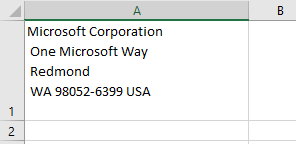VBA Programming | Code Generator does work for you!

### Split String into Worksheet Cells

You can copy the Split array into worksheet cells with just one command:

``````Sub CopyToRange()
'Create variables
Dim MyArray() As String, MyString As String
'Sample string with space delimiters
MyString = "One,Two,Three,Four,Five,Six"
'Use Split function to divide up the component parts of the string
MyArray = Split(MyString, ",")
'Copy the array into the worksheet
Range("A1:A" & UBound(MyArray) + 1).Value = WorksheetFunction.Transpose(MyArray)
End Sub
``````

When this code has been run, your worksheet will look like this: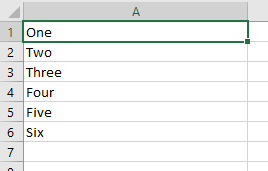### Creating a New Function to Allow Splitting from a Given Point

The Limit parameter in the Split function only allows you to specify an upper limit where you want the splitting to stop.  It always commences from the start of the string.

It would be very useful to have a similar function where you can specify the start point of the split within the string, and the number of splits that you want to see from that point onwards. It will also only extract the splits that you have specified into the array, rather than having an enormous string value as the last element in the array.

You can easily build a function (called SplitSlicer) yourself in VBA to do this:

``````Function SplitSlicer(Target As String, Del As String, Start As Integer, N As Integer)
'Create array variable
Dim MyArray() As String
'Capture the split using the start variable using the delimiter character
MyArray = Split(Target, Del, Start)
‘Check if the start parameter is greater than the number of splits – this can cause problems
If Start > UBound(MyArray) + 1 Then
‘Display error and exit the function
MsgBox "Start parameter is greater than number of splits available"
SplitSlicer = MyArray
Exit Function
End If
'Put the last array element into the string
Target = MyArray(UBound(MyArray))
'Split the string using N as the limit
MyArray = Split(Target, Del, N)
‘Check that the top limit is greater than zero as the code removes the last element
If UBound(MyArray) > 0 Then
'Use ReDim to remove the final element of the array
ReDim Preserve MyArray(UBound(MyArray) - 1)
End If
'Return the new array
SplitSlicer = MyArray
End Function
``````

This function is built with four parameters:

• Target – string – this is the input string that you want to split
• Del – string or non-printable character – this is the delimiter character that you use e.g. comma, colon
• Start – number – this is the start split for your slice
• N – number – this is the number of splits that you want to do within your slice

None of these parameters are optional or have default values, but you can work that into the code for the function if you wish to extend it further.

The function uses the Split function to create an array using the Start parameter as the Limit.  This means that the array elements will hold the splits up to the start parameter, but the remainder of the string will be the last element, and will not be split.

The last element in the array is transferred back to a string using the UBound function to determine which element this is.

The string is then split again into the array, using N as the limit variable.  This means that splits will be done for the string up to position N, after which the rest of the string will form the last element in the array.

The ReDim statement is used to remove the last element as we only want the specific elements left in the array.  Note that the Preserve parameter is used, otherwise all data in the array will be lost.

The new array is then returned to the code that it was called from.

Note that the code is ‘error proofed’.  Users will often do strange things that you did not consider.  For example, if they try using the function with the Start or N parameter greater than the available number of splits in the string, this is likely to cause the function to fail.

Code is included to check the Start value, and also to make sure that there is an element that can be removed when the ReDim statement is used on the array.

Here is the code to test the function:

``````Sub TestSplitSlicer()
'Create variables
Dim MyArray() As String, MyString As String
'Define sample string with comma delimiters
MyString = "One,Two,Three,Four,Five,Six,Seven,Eight,Nine,Ten"
'Use the Splitslicer function to define new array
MyArray = SplitSlicer(MyString, ",", 4, 3)
'Clear the active sheet
ActiveSheet.UsedRange.Clear
'Copy the array into the worksheet
Range("A1:A" & UBound(MyArray) + 1).Value = WorksheetFunction.Transpose(MyArray)
End Sub
``````

Run this code and your worksheet will look like this: